Next: An SEIR model with Up: APC/EEB/MOL 514 Tutorial 4: Previous: An SIS model with

# An SEIR model

We'll now consider the epidemic model from Seasonality and period-doubling bifurcations in an epidemic model'' by J.L. Aron and I.B. Schwartz, J. Theor. Biol. 110:665-679, 1984 in which the population consists of four groups:

•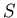is the fraction of susceptible individuals (those able to contract the disease),
•is the fraction of exposed individuals (those who have been infected but are not yet infectious),
•is the fraction of infective individuals (those capable of transmitting the disease),
•is the fraction of recovered individuals (those who have become immune).
Note that the variables give the fraction of individuals - that is, we have normalized them so that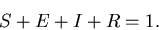(9)

Furthermore, suppose that
• There are equal birth and death rates,
•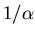is the mean latent period for the disease,
•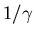is the mean infectious period,
• recovered individuals are permanently immune,
• the contact rate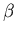may be a function of time.
This leads us to consider the following model: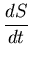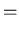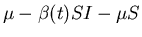(10)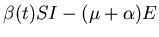(11)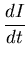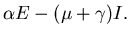(12)

The variableis determined from the other variables according to equation (9). When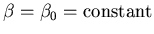, this is a three-dimensional autonomous system of ordinary differential equations, and is well understood. Defining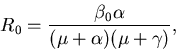(13)

it can be shown that forthe model has a fixed point with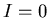which is unstable, and a fixed point withwhich is stable, etc. See Aron and Schwartz for more detail and references.

Ifdepends on time, we have a three-dimensional nonautonomous system, which can be converted to a four-dimensional autonomous system as was done above for the SIS model.Next: An SEIR model with Up: APC/EEB/MOL 514 Tutorial 4: Previous: An SIS model with
Jeffrey M. Moehlis 2002-10-14